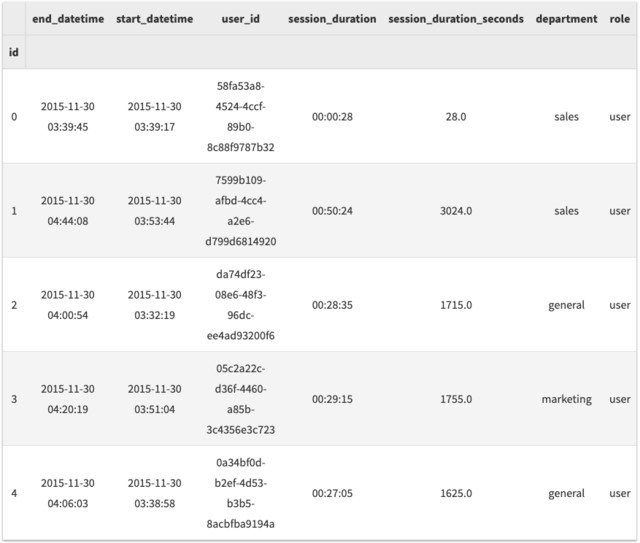【翻译】适用于产品经理的 Python 课程（三）——分组，汇总和总结

Posted by zhang on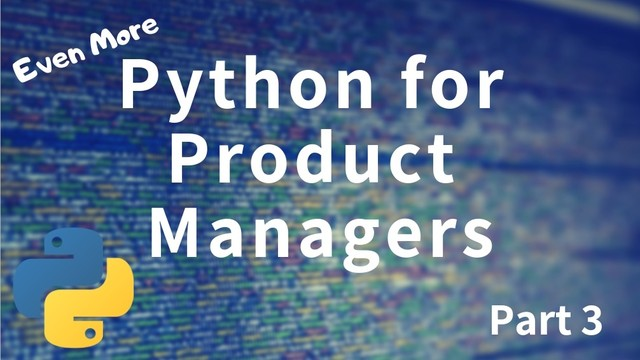关于这里的例子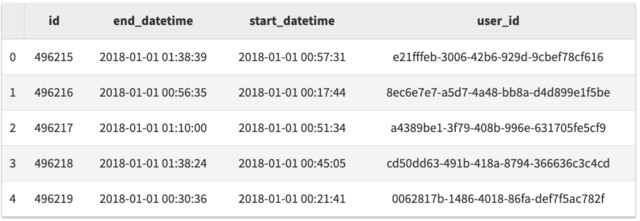数据分组

Pandas 有一个 groupby 的方法可以完成这个需求。我们可以指定指定按哪一列进行分组，以及应用聚合函数。常见的聚合函数有countmeanmedian

# 每一个用户有多少 sessions ?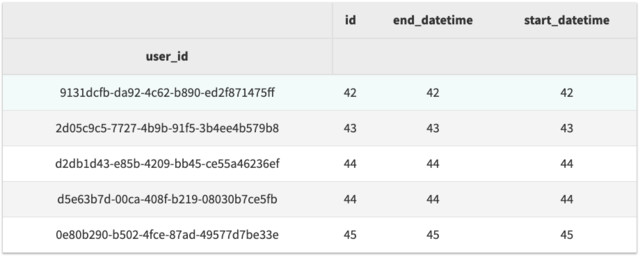# 每个月有多少 sessions
df.groupby(df.start_datetime.dt.month).agg('count')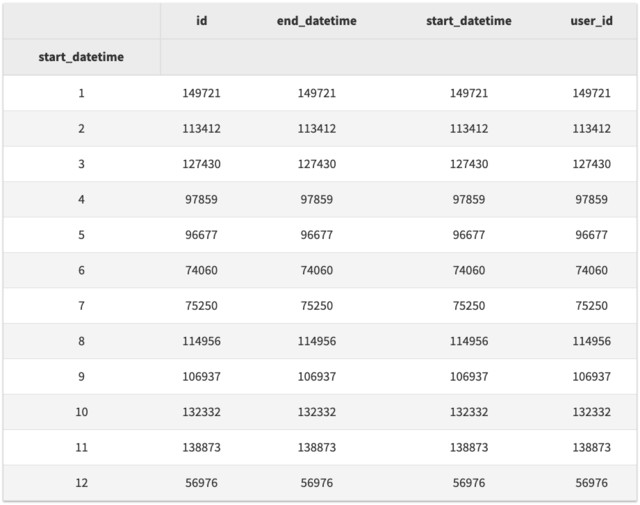#每一年的每个月有多少sessions
df.groupby([df.start_datetime.dt.year, df.start_datetime.dt.month]).agg('count').tail(n=24)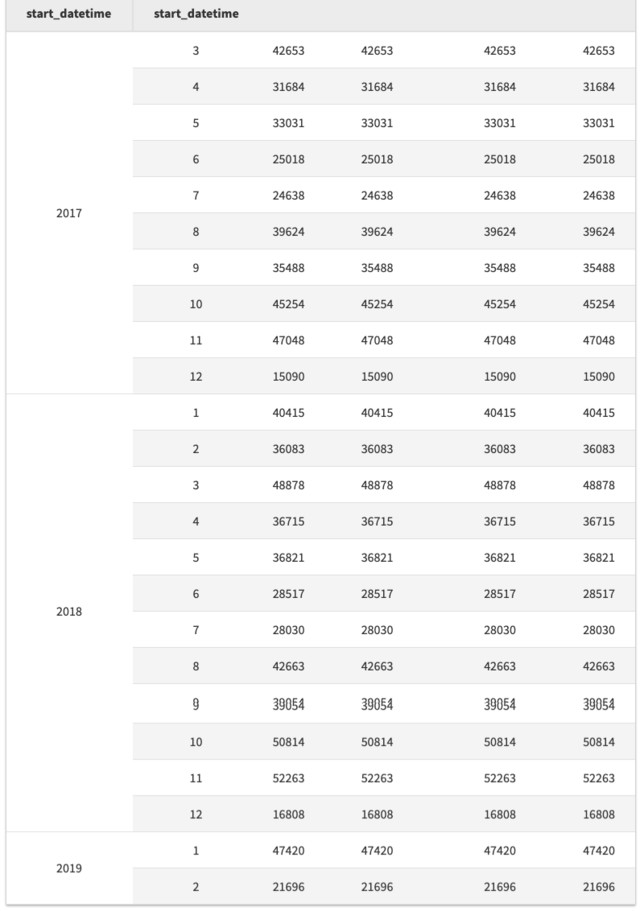# 计算每个 session 的长度
df['session_duration'] = df.end_datetime - df.start_datetime

# 现在看下 session 持续时间的一些统计数据
print(df['session_duration'].mean())
print(df['session_duration'].median())
print(df['session_duration'].min())
print(df['session_duration'].max())
0 days 00:34:02.443117
0 days 00:33:57
0 days 00:00:01
0 days 01:45:53

import numpy as np

# 以秒为单位计算 session 持续时间
df['session_duration_seconds'] = df['session_duration']/np.timedelta64(1,'s')

# 年和月的平均 session 持续时间（以秒为单位）
df[['session_duration_seconds']].groupby([df.start_datetime.dt.year, df.start_datetime.dt.month]).agg('mean').tail(n=12)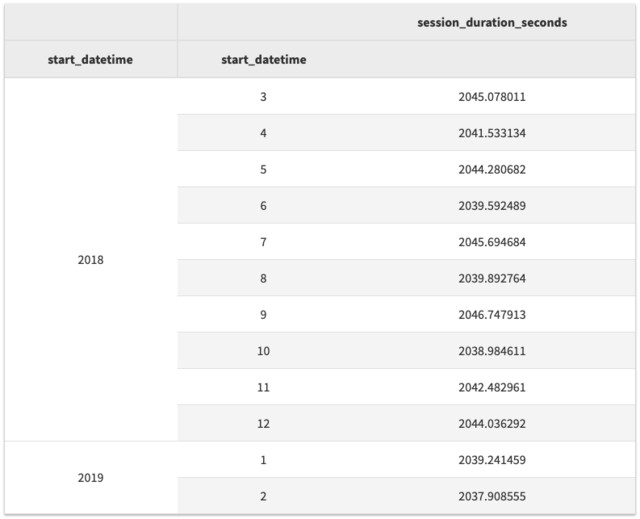# 以分钟为单位计算 session 持续时间
df['session_duration_seconds'] = df['session_duration']/np.timedelta64(1,'m')

数据描述

df['session_duration'].describe()
count                   1284483
mean     0 days 00:34:02.443117
std      0 days 00:14:49.732860
min             0 days 00:00:01
25%             0 days 00:23:51
50%             0 days 00:33:57
75%             0 days 00:44:06
max             0 days 01:45:53
Name: session_duration, dtype: object

将 PANDAS DATAFRAMES 集合在一起

• 他们的唯一 ID
• 角色（用户/管理员）
• 部门

# 从制表符分隔的文本文件加载用户数据
user_df = pd.read_csv('users.csv', sep='\t')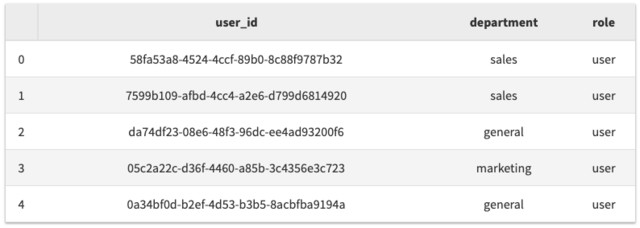# 将 sessions 数据将入用户数据
full_df = df.join(user_df, rsuffix='_user')
full_df.drop('user_id_user', axis=1, inplace=True)
full_df.set_index('id', inplace=True)